# ML Aggarwal Class 10 Solutions for ICSE Maths Chapter 3 Shares and Dividends Ex 3

## ML Aggarwal Class 10 Solutions for ICSE Maths Chapter 3 Shares and Dividends Ex 3

ML Aggarwal Class 10 Solutions for ICSE Maths Chapter 3 Shares and Dividends Ex 3

Question 1.
Find the dividend received on 60 shares of Rs, 20 each if 9% dividend is declared.
Solution: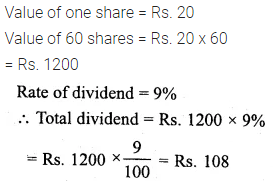Question 2.
A company declares 8 per cent dividend to the shareholders. If a man receives Rs. 2840 as his dividend, find the nominal value of his shares.
Solution: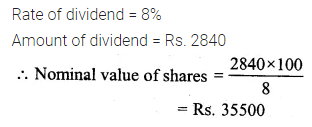Question 3.
A man buys 200 ten-rupee shares at Rs 12.50 each and receives a dividend of 8%. Find the amount invested by him and the dividend received by him in cash.
Solution: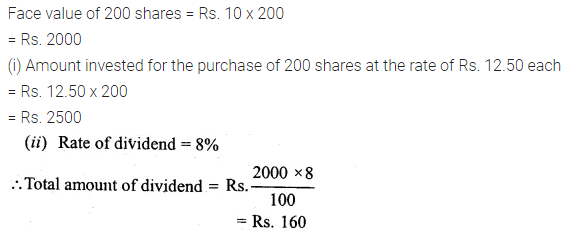Question 4.
Find the market price of 5% share when a person gets a dividend of Rs 65 by investing Rs. 1430.
Solution: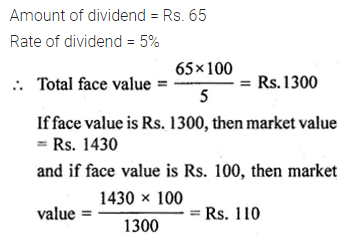Question 5.
Salman buys 50 shares of face value Rs 100 available at Rs 132.
(i) What is his investment?
(ii) If the dividend is 7.5% p.a., what will be his annual income?
(iii) If he wants to increase his annual income by Rs 150, how many extra shares should he
Solution: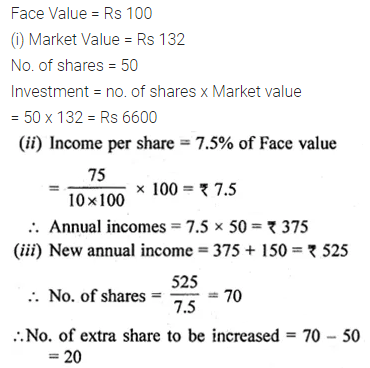Question 6.
A lady holds 1800, Rs. 100 shares of a company that pays 15% dividend annually. Calculate her annual dividend. If she had bought these shares at 40% premium, what percentage return does she get on her investment? Give your answer to the nearest integer.
Solution: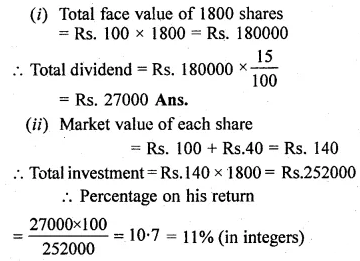Question 7.
What sum should a person invest in Rs 25 shares, selling at Rs 36, to obtain an income of Rs 720, if the dividend declared is 12%? Also, find the percentage return on his income.
(i) The number of shares bought by him.
(ii) The percentage return on his income.
Solution: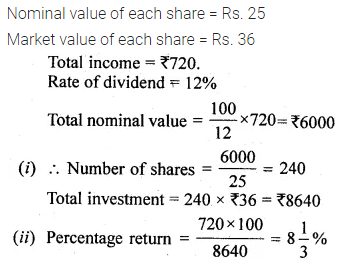Question 8.
Ashok invests Rs 26400 on 12% Rs 25 shares of a company. If he receives a dividend of Rs 2475, find:
(i) the number of shares he bought.
(ii) the market value of each share. (2016)
Solution: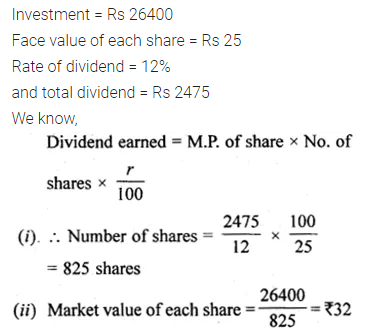Question 9.
Amit Kumar invests Rs 36,000 in buying Rs 100 shares at Rs 20 premium. The dividend is 15% per annum. Find :
(i) The number of shares he buys
(ii) His yearly dividend
(iii) The percentage return on his investment.
Solution:Question 10.
Mr Tiwari invested Rs 29,040 in 15% Rs 100 shares at a premium of 20%. Calculate:
(i) The number of shares bought by Mr Tiwari.
(ii) Mr Tiwari’s income from the investment.
(iii) The percentage return on his investment.
Solution:Question 11.
A man buys shares at the par value of Rs 10 yielding 8% dividend at the end of a year. Find the number of shares bought if he receives a dividend of Rs 300.
Solution:Question 12.
A man invests Rs 8800 on buying shares of the face value of rupees hundred each at a premium of 10%. If he earns Rs 1200 at the end of the year as a dividend, find:
(i) the number of shares he has in the company.
(ii) the dividend percentage per share.
Solution:Question 13.
A man invested Rs. 45000 in 15% Rs. 100 shares quoted at Rs. 125. When the market value of these shares rose to Rs. 140, he sold some shares, just enough to raise Rs. 8400. Calculate :
(i) the number of shares he still holds. (2004)
(ii) the dividend due to him on these shares.
Solution:Question 14.
A company pays a dividend of 15% on its ten-rupee shares from which it deducts tax at the rate of 22%. Find the annual income of a man, who owns one thousand shares of this company.
Solution:Question 15.
Ajay owns 560 shares of a company. The face value of each share is Rs. 25. The company declares a dividend of 9%. Calculate.
(i) the dividend that Ajay will get.
(ii) the rate of interest, on his investment, if Ajay has paid Rs. 30 for each share. (2007)
Solution:Question 16.
A company with 10000 shares of the nominal value of Rs. 100 declares an annual dividend of 8% to the shareholders.
(i) Calculate the total amount of dividend paid by the company.
(ii) Ramesh bought 90 shares of the company at Rs. 150 per share.
Calculate the dividend he received and the percentage return on his investment. (1994)
Solution:Question 17.
A company with 4000 shares of the nominal value of Rs. 110 declares an annual dividend of 15%. Calculate :
(i) the total amount of dividend paid by the company,
(ii) the annual income of Shah Rukh who holds 88 shares in the company,
(iii) if he received only 10% on his investment, find the price Shah Rukh paid for each share. (2008)
Solution:Question 18.
By investing in Rs. 7500 in a company paying 10 per cent dividend, an income of Rs. 500 is received. What price is paid for each Rs 100 share
Solution:Question 19.
A man invests Rs. 8000 in a company paying 8% dividend when a share of the face value of Rs. 100 is selling at Rs. 60 premium,
(i) What is his annual income,
(ii) What per cent does he get on his money?
Solution:Question 20.
A man buys 400 ten-rupee shares at a premium of Rs. 2.50 on each share. If the rate of dividend is 8%, Find,
(i) his investment
(iii) yield.
Solution:Question 21.
A man invests Rs. 10400 in 6% shares at Rs. 104 and Rs. 11440 in 10.4% shares at Rs. 143. How much income would he get in all?
Solution:Question 22.
Two companies have shares of 7% at Rs. 116 and 9% at Rs. 145 respectively. In which of the shares would the investment be more profitable?
Solution:Question 23.
Which is a better investment: 6% Rs. 100 shares at Rs. 120 or 8% Rs. 10 shares at Rs. 15
Solution:Question 24.
A man invests Rs -10080 in 6% hundred- rupee shares at Rs. 112. Find his annual income. When the shares fall to Rs. 96 he sells out the shares and invests the proceeds in 10% ten-rupee shares at Rs. 8. Find the change in his annual income.
Solution: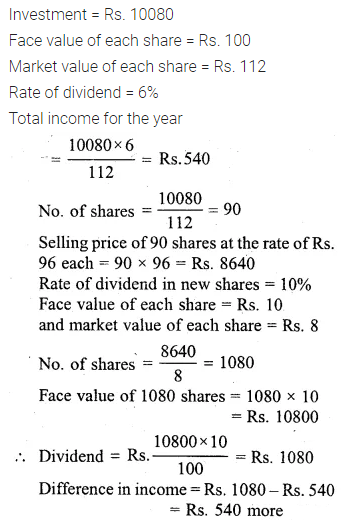Question 25.
A man bought 360 ten-rupee shares paying 12% per annum. He sold them when the price rose to Rs. 21 and invested the proceeds in five-rupee shares paying $$4 \frac { 1 }{ 2 }$$ % per annum at Rs. 3.5 per share. Find the annual change in his income.
Solution:Question 26.
A person invests Rs. 4368 and buys certain hundred-rupee shares at 91. He sells out shares worth Rs. 2400 when they have t risen to 95 and the remainder when they have fallen to 85. Find the gain or loss on the total transaction,
Solution:Question 27.
By purchasing Rs. 50 gas shares for Rs. 80 each, a man gets a 4% profit on his investment. What rate per cent is company paying? What is his dividend if he buys 200 shares?
Solution:Question 28.
Rs. 100 shares of a company are sold at a discount of Rs. 20. If the return on the investment is 15%. Find the rate of dividend declared
Solution: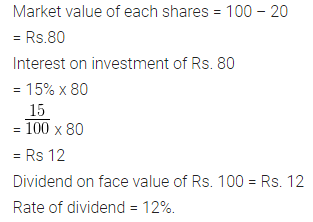Question 29.
A company declared a dividend of 14%. Find the tire market value of Rs. 50 shares if the return on the investment was 10%.
Solution:Question 30.
At what price should a 6.25% Rs. 100 shares are quoted when the money is worth 5%?
Solution:Question 31.
At what price should a 6.25% Rs. 50 share be quoted when the money is worth 10%?
Solution:Question 32.
A company with 10000 shares of Rs. 100 each, declares an annual dividend of 5%.
(i) What is the total amount of dividend paid by the company?
(ii) What would be the annual income of a man, who has 72 shares, in the company?
(iii) If he received only 4% on his investment, find the price he paid for each share. (1998)
Solution:Question 33.
A man sold some Rs. 100 shares paying 10% dividend at a discount of 25% and invested the proceeds in Rs. 100 shares paying a 16% dividend quoted at Rs. 80 and thus increased his income by Rs. 2000. Find the number of shares sold by him.
Solution:Question 34.
By selling at Rs. 77, some $$2 \frac { 1 }{ 4 }$$ % shares of face value Rs. 100, and investing the proceeds in 6% shares of face value Rs. 100, selling at 110, a person increased his income by Rs, 117 per annum. How many shares did he sell?
Solution:Question 35.
A man invests Rs. 6750, partly in shares of 6% at Rs. 140 and partly in shares of 5% at Rs. 125. If his total income is Rs. 280, how much has he invested in each?
Solution:Question 36.
Divide Rs. 20304 into two parts such that if one part is invested in 9% Rs. 50 shares at 8% premium and the other part are invested in 8% Rs. 25 shares at 8% discount, then the annual incomes from both the investment are equal
Solution: